# Fault Calculation - Per Unit System

By on

Symbol   Definition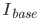- per unit method current base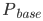- per unit method power base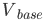- per unit method voltage base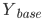- per unit method admittance base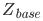- per unit method impedance base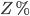- percentage impedance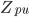- per unit impedance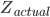- actual impedance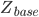- per unit base impedance

Per unit fault calculations is a method whereby system impedances and quantities are normalised across different voltage levels to a common base.  By removing the impact of varying voltages, the necessary calculations are simplified.

To use the per unit method, we normalise all the system impedances (and admittances) within the network under consideration to a common base.  These normalised impedances are know as per unit impedances.  Any per unit impedance will have the same value on both the primary and secondary of a transformer and is independent of voltage level.

A network of per unit impedances can then be solved using standard network analysis (see the example).  From this fault level can be readily determined.

In applying the per unit method, the first step is to select an arbitrary voltage (Vbase) and power (Pbase) base.

Tip: while the base power and voltage be any value, typically it would make sense to select values related to the system under construction (for example 11 kV and 20 MVA may be appropriate for a distribution type system)

## Per Unit Method

Having selected a base power and voltage, the base per unit values of impedance, admittance and current can be calculated from:

Per Unit Single Phase Three Phase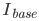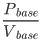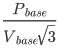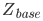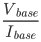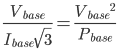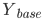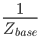Dividing a system element by it’s per-unit base value gives the per-unit value of the element, for example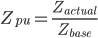Some times per-unit values are available for a given base kV, but the problem being solved is using a different base.  In this instance it is possible to convert the unit:Fault calculation problems typically deal wit power sources, generators, transformers and system impedances.  Per-unit values for these elements can be quickly derived from:

Element Per-Unit Value
Source impedance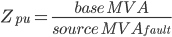Generators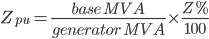Transformers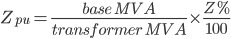Impedances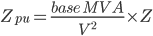, where V is in kV

#### Example - calculating per unit values

Consider a system of source impedance 4.48 Ω connected to a 20 MVA transformer (11/0.4 kV) at 6% impedance.  We want to find the fault level at the transformer secondary.

Selecting Pbase as 20 MVA and Vbase as 11 kV and using the above equations:and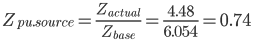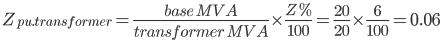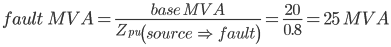the Line-Neutral voltage on the secondary of the transformer is 0.4/√3 = 0.230 kV, giving: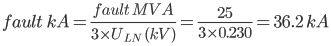### Three Phase Fault Example

Per unit analysis can be used to calculate system three phase fault levels and the current distributions.  To gain a better understanding, it is worth running through the typical steps required to solve a fault calculation problem.

Given the system single line diagram, construct and simplify the per unit impedance diagram.

The fault level at the point under consideration is given by: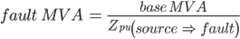Where Zpu, is the total impedance between the source and the fault.

Fault flow through parallel branches is given by the ratio of impedances. As illustrated this can enable fault flows to be found through each branch.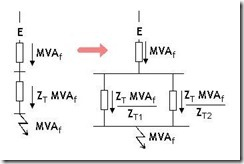Having calculated the fault flow in each branch, it is then relatively simple to find the current distribution using: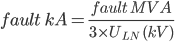## Related Notes

More interesting Notes:Steven McFadyen

Steven has over twenty five years experience working on some of the largest construction projects. He has a deep technical understanding of electrical engineering and is keen to share this knowledge. About the author

#### View 3 Comments (old system)

1.FELIX JR. says:
5/21/2013 12:52 PM

greetings !, hello !

this article which you have shared is a good explanation for short circuit current calculations using the P.U. Method based on a given kVA base, as this is the old school way (i.e. during my college days and my early prof. work with my employer).

But how do you find and comment on the IEC-60909 way of using the ohmic method and also that of the MVA method, alternative ? So, which of the three (3) are more easy to use and accurate ?

felix jr.

2.gilly1 says:
5/29/2013 11:41 PM

Just wondering which method do you think the most accurate for calculating single phase-earth faults, the Ohmic Method or Symmetrical Component Method.
The Symm. Comp. method is quite complex and while a lot of people use it to solve complex types of unbalanced faults it is not real in the sense that there aren't really 3 currents flowing at once : Pos, Neg and Zero sequence currents, whereas the Ohmic Method is based in reality with real impedances???

3.Steven says:
5/30/2013 8:03 AM

My opinion is that symmetrical components would be more accurate (especially for any unbalanced faults like phase to earth).

If your looking for more practical guidance on using symmetrical components, then I would reefer to IEC 60909. This standard is also implemented in many software programs.

Comments are closed for this post:
• have a question or need help, please use our Questions Section
• spotted an error or have additional info that you think should be in this post, feel free to Contact Us

Capacitor Theory

Capacitors are widely used in electrical engineering for functions such as energy storage, power factor correction, voltage compensation and many others...

Voltage Levels – Confused?

I was having a conversation the other day about voltage levels.  While everyone was in agreement that low voltage was 1000 V and less, there was more confusion...

Maximum Demand for Buildings

Estimating maximum demand is a topic frequently discussed. Working out how much power to allow for a building can be very subjective . Allowing too much...

Cable Sizing Software

When sizing cables nearly, everyone uses some form of software. This ranges from homespun spreadsheets to complex network analyses software. Each has its...

Understanding LV Circuit Breaker Fault Ratings

I think this post is going to be helpful to several of our readers. While the IEC low voltage circuit breaker Standard [IEC 60947-2, Low voltage switchgear...

ABB Technical Guides - Motor Operation

ABB has produced a range of technical guides that offer concise explanations of the major technologies and technical issues in low voltage AC drives. ...

The dc resistance of conductors

This is the first of two posts on the resistance of conductors. In the next post I will look at the ac resistance, including skin effect and we deal with...

Maxwell's Equations - Introduction

Maxwell's Equations are a set of fundamental relationships, which govern how electric and magnetic fields interact. The equations explain how these fields...

Equipment Verification (to IEC Standards)

One of the requirements to ensuring that everything works is to have equipment selected, manufactured and verified [tested] to IEC standards. Not all equipment...

Understanding Motor Duty Rating

One of the comments on my Motor Starting Series was asking for something on duty cycles. Here it is. As a purchaser of a motor, you have responsibility...## Have some knowledge to share

If you have some expert knowledge or experience, why not consider sharing this with our community.

By writing an electrical note, you will be educating our users and at the same time promoting your expertise within the engineering community.

To get started and understand our policy, you can read our How to Write an Electrical Note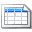Click here to view the notes list in a table formatClick here to view the notes indexed by tag